MNIST

Database of handwritten digits commonly used for training image processing systems

Originator: Yann LeCun, Corinna Cortes, and Christopher J.C. Burges

Examples

Basic Examples

Retrieve the ResourceObject:

 In:=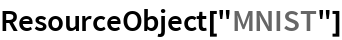Out=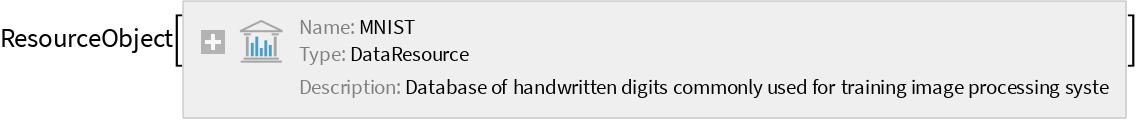View the data:

 In:=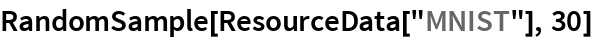Out=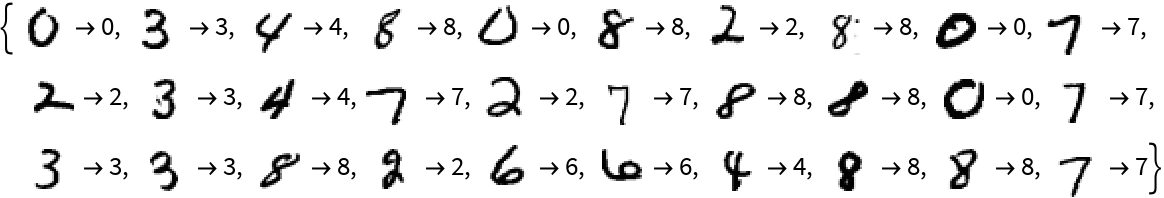Visualization

Create a 3D image by stacking digit images:

 In:=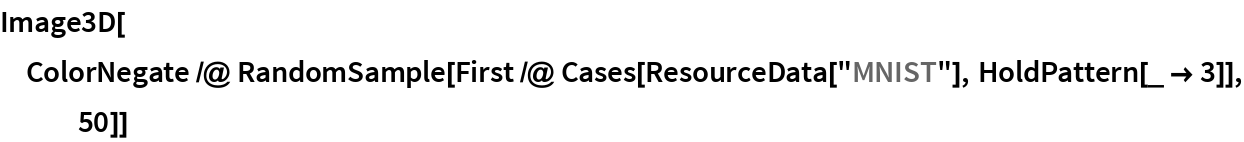Out=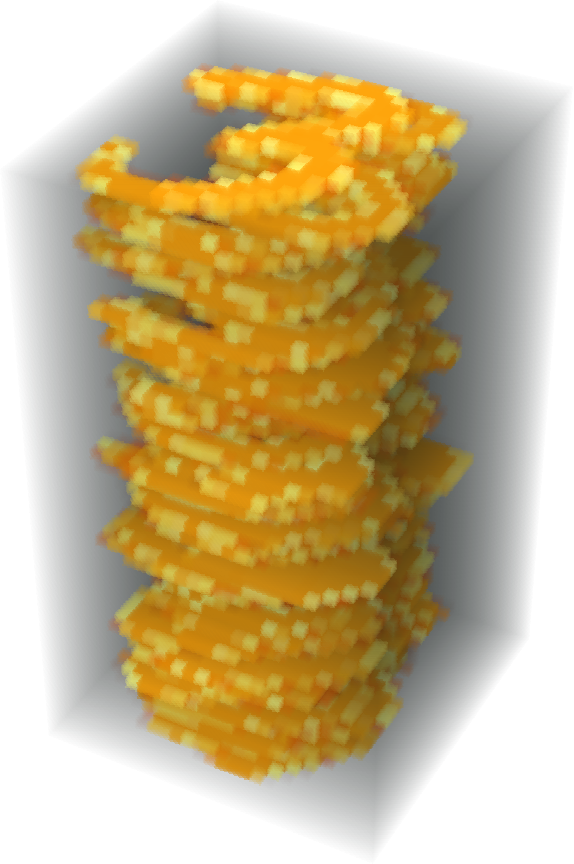Analysis

Train and test a classifier:

 In:=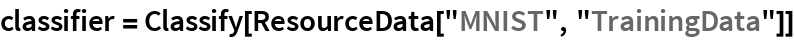Out=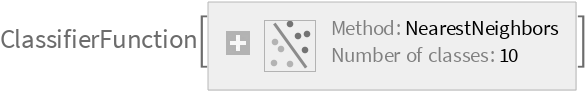In:=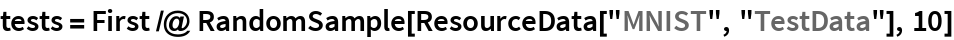Out=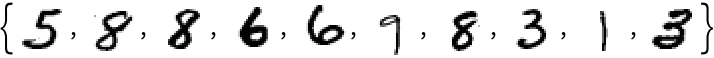In:=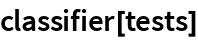Out=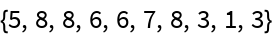Wolfram Research, "MNIST" from the Wolfram Data Repository (2016) https://doi.org/10.24097/wolfram.62081.data